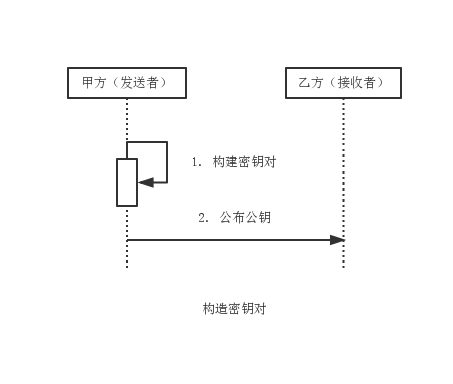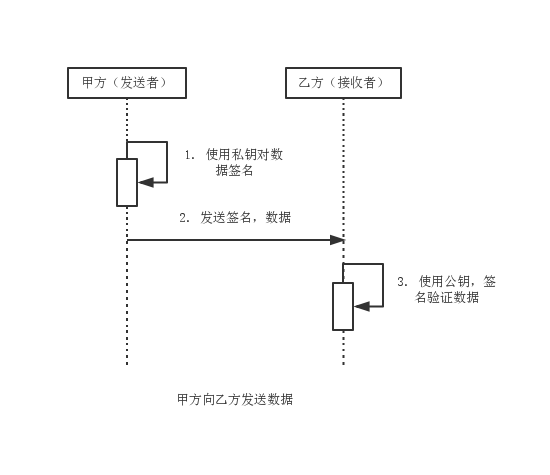# rsa签名算法c语言,数字签名算法rsaRSA

RSA数字签名算法源于RSA公钥密码算法的思想，将RSA公钥密码算法按照数字签名的方式运用。RSA数字签名算法是迄今为止应用最为广泛的数字签名算法。 RSA数字签名算法的实现如RSA加密算法一致。RSA数字签名算法主要可分为MD系列和SHA系列。

MD系列主要包括：MD2withRSA和MD5withRSA。

SHA系列主要包括：SHA1withRSA，SHA224withRSA，�SHA256withRSA，SHA384withRSA，SHA512withRSA。

Java 6提供了MD2withRSA，MD5withRSA，SHA1withRSA支持，其他四中SHA算法第三方加密组建包Bouncy Castle提供支持。

1)消息发送者产生一个密钥对(私钥+公钥)，然后将公钥发送给消息接收者

2)消息发送者使用消息摘要算法对原文进行加密(加密后的密文称作摘要)

3)消息发送者将上述的摘要使用私钥加密得到密文--这个过程就被称作签名处理，得到的密文就被称作签名(注意，这个签名是名词)

4)消息发送者将原文与密文发给消息接收者

5)消息接收者使用公钥对密文(即签名)进行解密，得到摘要值content1

6)消息接收者使用与消息发送者相同的消息摘要算法对原文进行加密，得到摘要值content2

7)比较content1是不是与content2相等，若相等，则说明消息没有被篡改(消息完整性)，也说明消息却是来源于上述的消息发送方(因为其他人是无法伪造签名的，这就完成了“抗否认性”和“认证消息来源”)

RSA: 加密原理

RSA-Algorithm

RSA算法演示程序，仅供了解RSA算法实现原理

RSA算法原理

N = P * Q

M = (P - 1) * (Q - 1)

E是公钥，负责加密

D是私钥，负责解密

N负责公钥和私钥之间的联系

(X ^ E) % N = Y

(Y ^ D) % N = X

RSA本身算法的核心思想还是比较简单的，加密、解密算法的区别也只是在乘方取模部分使用的数字有所区别而已

N和E可以公开给任何人加密使用，但是只有掌握密钥D的人才可以解密，即使加密者自己也无法解密

P = 11;

Q = 13;

N = 143;

M = 120;

E = 89;

D = 209;

using namespace std;

int Plaintext;//明文

long long Ciphertext;//密文

int n, e = 0, d;

//二进制转换

int BianaryTransform(int num, int bin_num[])

{

int i = 0, mod = 0;

//转换为二进制，逆向暂存temp[]数组中

while(num != 0)

{

mod = num%2;

bin_num[i] = mod;

num = num/2;

i++;

}

//返回二进制数的位数

return i;

}

//反复平方求幂

long long Modular_Exonentiation(long long a, int b, int n)

{

int c = 0, bin_num;

long long d = 1;

int k = BianaryTransform(b, bin_num)-1;

for(int i = k; i >= 0; i--)

{

c = 2*c;

d = (d*d)%n;

if(bin_num[i] == 1)

{

c = c + 1;

d = (d*a)%n;

}

}

return d;

}

//生成1000以内素数

{

int c = 0, vis;

memset(vis, 0, sizeof(vis));

for(int i = 2; i <= 1000; i++)if(!vis[i])

{

prime[c++] = i;

for(int j = i*i; j <= 1000; j+=i)

vis[j] = 1;

}

return c;

}

//欧几里得扩展算法

int Exgcd(int m,int n,int &x)

{

int x1,y1,x0,y0, y;

x0=1; y0=0;

x1=0; y1=1;

x=0; y=1;

int r=m%n;

int q=(m-r)/n;

while(r)

{

x=x0-q*x1; y=y0-q*y1;

x0=x1; y0=y1;

x1=x; y1=y;

m=n; n=r; r=m%n;

q=(m-r)/n;

}

return n;

}

//RSA初始化

void RSA_Initialize()

{

//取出1000内素数保存在prime[]数组中

int prime;

//随机取两个素数p,q

srand((unsigned)time(NULL));

int ranNum1 = rand()%count_Prime;

int ranNum2 = rand()%count_Prime;

int p = prime[ranNum1], q = prime[ranNum2];

n = p*q;

int On = (p-1)*(q-1);

//用欧几里德扩展算法求e,d

for(int j = 3; j < On; j+=1331)

{

int gcd = Exgcd(j, On, d);

if( gcd == 1 && d > 0)

{

e = j;

break;

}

}

}

//RSA加密

void RSA_Encrypt()

{

cout<

';

cout<

'<

';

int i = 0;

for(i = 0; i < 100; i++)

Ciphertext[i] = Modular_Exonentiation(Plaintext[i], e, n);

cout<

';

for(i = 0; i < 100; i++)

cout<

cout<

'<

';

}

//RSA解密

void RSA_Decrypt()

{

int i = 0;

for(i = 0; i < 100; i++)

Ciphertext[i] = Modular_Exonentiation(Ciphertext[i], d, n);

cout<

';

for(i = 0; i < 100; i++)

cout<

cout<

'<

';

}

//算法初始化

void Initialize()

{

int i;

srand((unsigned)time(NULL));

for(i = 0; i < 100; i++)

Plaintext[i] = rand()%1000;

cout<

';

for(i = 0; i < 100; i++)

cout<

cout<

'<

';

}

int main()

{

Initialize();

while(!e)

RSA_Initialize();

RSA_Encrypt();

RSA_Decrypt();

return 0;

}

Ref：

http://wzhong.logdown.com/posts/234502-rsa-c

https://github.com/liufan321/RSA-Algorithm

https://github.com/LexHsu/Summary/blob/master/02-Algorithm/book/5.1-rsa.md#数字签名算法消息传递模型

12-23
06-25
05-28
01-011988
05-242511
05-251253
05-14906
05-24541
11-24946
07-183062
12-041704
07-23
02-02888
09-243万+
10-23190
12-021276
01-292673

### “相关推荐”对你有帮助么？

•非常没帮助
•没帮助
•一般
•有帮助
•非常有帮助被折叠的  条评论 为什么被折叠?到【灌水乐园】发言点击重新获取扫码支付1.余额是钱包充值的虚拟货币，按照1:1的比例进行支付金额的抵扣。
2.余额无法直接购买下载，可以购买VIP、C币套餐、付费专栏及课程。余额充值